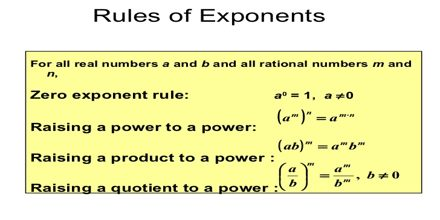Mathematic

# Rules for Rational ExponentsPrime objective of this lecture is to present on rules for Rational Exponents. It is the reciprocal of 16/25 with a positive exponent. So it is the square root of 25/16, which is 5/4, raised to the 3rd power: 125/64. An exponent may now be any rational number. Rational exponents u, v will obey the usual rules. Until this point we have only had exponents that are integers, so it is time to introduce two new rules that deal with rational (or fractional) exponents. These rules will help to simplify radicals with different indices by rewriting the problem with rational exponents.